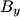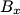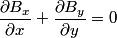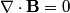## Solution to 1986 Problem 79

 (A) Assume the upward direction is y and the horizontal direction is x. Then$B_y$ = 0 everywhere and$B_x$ does not change as you go in the x direction ($B_x$ does, however, change as you go in the y direction). Thus,\begin{align*}\frac{\partial B_x}{\partial x} + \frac{\partial B_y}{\partial y} = 0\end{align*}So, the equation is obeyed. (B) shows a constant magnetic field, which is clearly divergenceless. (C) shows the magnetic field due to a current loop, which is clearly possible. (D) shows the electric field due to a point charge (i.e. an electric monopole); since magnetic monopole's do not exist (which is equivalent to the state$\nabla \cdot \mathbf{B} = 0$), this field configuration is not possible. Thus, answer (D) is correct. (E) is the magnetic field around a wire, which is clearly possible.